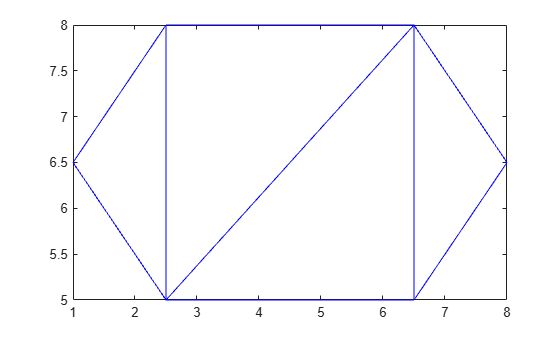# triangulation

Triangulation in 2-D or 3-D

## Description

Use `triangulation` to create an in-memory representation of any 2-D or 3-D triangulation data that is in matrix format, such as the matrix output from the `delaunay` function or other software tools. When your data is represented using `triangulation`, you can perform topological and geometric queries, which you can use to develop geometric algorithms. For example, you can find the triangles or tetrahedra attached to a vertex, those that share an edge, their circumcenters, and other features.

## Creation

To create a `triangulation` object, use the `triangulation` function with input arguments that define the triangulation's points and connectivity.

### Syntax

``TR = triangulation(T,P)``
``TR = triangulation(T,x,y)``
``TR = triangulation(T,x,y,z)``

### Description

example

````TR = triangulation(T,P)` creates a 2-D or 3-D triangulation representation using the triangulation connectivity list `T` and the points in matrix `P`.```
````TR = triangulation(T,x,y)` creates a 2-D triangulation representation with the point coordinates specified as column vectors `x` and `y`.```
````TR = triangulation(T,x,y,z)` creates a 3-D triangulation representation with the point coordinates specified as column vectors `x`, `y`, and `z`. ```

### Input Arguments

expand all

Triangulation connectivity list, specified as an `m`-by-`n` matrix, where `m` is the number of triangles or tetrahedra, and `n` is the number of vertices per triangle or tetrahedron. Each row of `T` contains the vertex IDs that define a triangle or tetrahedron. The vertex IDs are the row numbers of the input points. The ID of a triangle or tetrahedron in the triangulation is the corresponding row number in `T`.

Points, specified as a matrix whose columns are the x-coordinates, y-coordinates, and (possibly) `z`-coordinates of the triangulation points. The row numbers of `P` are the vertex IDs in the triangulation.

x-coordinates of triangulation points, specified as a column vector.

y-coordinates of triangulation points, specified as a column vector.

z-coordinates of triangulation points, specified as a column vector.

## Properties

expand all

Triangulation points, represented as a matrix with the following characteristics:

• Each row in `TR.Points` contains the coordinates of a vertex.

• Each row number of `TR.Points` is a vertex ID.

Triangulation connectivity list, represented as a matrix with the following characteristics:

• Each element in `TR.ConnectivityList` is a vertex ID.

• Each row represents a triangle or tetrahedron in the triangulation.

• Each row number of `TR.ConnectivityList` is a triangle or tetrahedron ID.

## Object Functions

 `barycentricToCartesian` Convert coordinates from barycentric to Cartesian `cartesianToBarycentric` Convert coordinates from Cartesian to barycentric `circumcenter` Circumcenter of triangle or tetrahedron `edgeAttachments` Triangles or tetrahedra attached to specified edge `edges` Triangulation edges `faceNormal` Triangulation unit normal vectors `featureEdges` Sharp edges of surface triangulation `freeBoundary` Free boundary facets `incenter` Incenter of triangulation elements `isConnected` Test if two vertices are connected by an edge `nearestNeighbor` Vertex closest to specified point `neighbors` Triangle or tetrahedron neighbors `pointLocation` Triangle or tetrahedron enclosing point `size` Size of triangulation connectivity list `vertexAttachments` Triangles or tetrahedra attached to vertex `vertexNormal` Triangulation vertex normal

## Examples

collapse all

Define and plot the points in a 2-D triangulation.

```P = [ 2.5 8.0 6.5 8.0 2.5 5.0 6.5 5.0 1.0 6.5 8.0 6.5];```

Define the triangulation connectivity list.

```T = [5 3 1; 3 2 1; 3 4 2; 4 6 2];```

Create and plot the triangulation representation.

`TR = triangulation(T,P)`
```TR = triangulation with properties: Points: [6x2 double] ConnectivityList: [4x3 double] ```
`triplot(TR)`Examine the coordinates of the vertices of the first triangle.

`TR.Points(TR.ConnectivityList(1,:),:)`
```ans = 3×2 1.0000 6.5000 2.5000 5.0000 2.5000 8.0000 ```

## Version History

Introduced in R2013a